Decimal range decomposition

Decimal range decomposition is a standard technique used to simplify problems that involve the digits of ranges (i.e, intervals) of natural numbers.

Informal idea

Consider the following problem: given natural number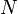$N$, how many of the natural numbers between 1 and$N$, inclusive, have a non-ascending sequence of digits? That is, each digit is less than or equal to the digit on its left, if any. For example, 100 and 321 qualify, but 101 and 123 do not. Assume that the naive algorithm is not efficient enough as$N$ can have up to, say, 15 digits, so performing$N$ loop iterations is out of the question.

It is hard to find an explicit formula for this quantity, but notice that there are some ranges of natural numbers for which we can write down a closed form. For example, if the range, instead of being from 1 to$N$, were to be from 35000 to 35999, we know at a glance that the answer is 0, since any number starting with the prefix "35" does not have a descending digit sequence. If instead the range were 53000 to 53999, the question becomes: in how many ways can we fill in the digits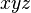$xyz$ so that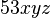$53xyz$ has a descending digit sequence? Well, clearly, each of the digits$xyz$ can only be 0, 1, 2, or 3. How many ways are there to fill in three slots, each with one of four possible digits, so that the resulting sequence is descending? This can easily be calculated as the binomial coefficient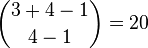$\binom{3 + 4 - 1}{4 - 1} = 20$.

So it is easy to see that if we are given a range of the form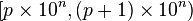$[p \times 10^n, (p+1) \times 10^n)$ with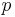$p$ nonzero (which we'll call an elementary range), then the number of integers in this range with descending digits is either zero if the prefix$p$ itself does not have descending digits, or else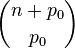$\binom{n + p_0}{p_0}$ where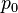$p_0$ is the last digit of the prefix$p$. For example, the range 53000 to 53999 (inclusive) is represented by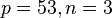$p = 53, n = 3$.

If the actual range given is from 1 to$N$, then we can try to decompose this range into elementary ranges, compute the number of integers with descending digits in each elementary range as a simple binomial coefficient, and then sum the counts obtained in order to get the final answer. For example, if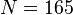$N = 165$, then we can decompose the range [1, 123] as the disjoint union of the following ranges: [1, 2), [2, 3), [3, 4), [4, 5), [5, 6), [6, 7), [7, 8), [8, 9), [9, 10), [10, 20), [20, 30), [30, 40), [40, 50), [50, 60), [60, 70), [70, 80), [80, 90), [90, 100), [100, 110), [110, 120), [120, 130), [130, 140), [140, 150), [150, 160), [160, 161), [161, 162), [162, 163), [163, 164), [164, 165), [165, 166). Note that each range is elementary: each range corresponds to the entire set of natural numbers corresponding to some fixed prefix with some fixed number of arbitrary digits following.

Formal definition

The decimal range decomposition of the interval of natural numbers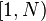$[1, N)$ is the disjoint union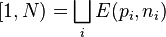$[1, N) = \bigsqcup_i E(p_i, n_i)$

where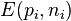$E(p_i, n_i)$ denotes an elementary interval, with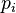$p_i$ is a positive integer and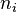$n_i$ a nonnegative integer: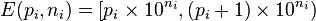$E(p_i, n_i) = [p_i \times 10^{n_i}, (p_i+1) \times 10^{n_i})$

and the set of elementary intervals used is as small as possible.

Algorithm

To obtain the decimal range decomposition of$[1, N)$, there are two stages. In the first stage, we count all integers that have fewer digits than$N$. That is, we decompose the range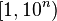$[1, 10^n)$, where$n$ is the largest possible integer such that this range is contained within$[1, N)$. That is,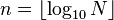$n = \lfloor \log_{10} N \rfloor$. In the second stage, we count up to$N$ by advancing the leftmost digit, then the next-to-leftmost, and so on, "honing in" on the digit sequence of$N$.

First stage

Compute$n = \lfloor \log_{10} N \rfloor$. Then decompose the range$[1, 10^n)$ as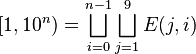$[1, 10^n) = \bigsqcup_{i=0}^{n-1} \bigsqcup_{j=1}^9 E(j, i)$

Second stage

It remains to decompose the range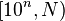$[10^n, N)$, where$N$ has the same number of digits as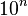$10^n$. Let the digit sequence of$N$ be denoted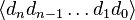$\langle d_n d_{n-1} \ldots d_1 d_0 \rangle$. Then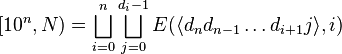$[10^n, N) = \bigsqcup_{i=0}^n \bigsqcup_{j=0}^{d_i-1} E(\langle d_n d_{n-1} \ldots d_{i+1} j\rangle, i)$

Implementation (C++)

#include <vector>
#include <utility>
std::vector<std::pair<long long, int>> drd(long long N) {
std::vector<std::pair<long long, int>> res;
int exponent = 0;
long long pwr = 1;
// stage 1
while (N/pwr >= 10ll) {
for (int d = 1; d <= 9; d++) {
res.push_back({d, exponent});
}
pwr *= 10; exponent++;
}
// stage 2
long long l = pwr;
long long p = 1;
while (l < N) {
if (l + pwr <= N) {
res.push_back({p, exponent});
l += pwr;
p++;
} else {
pwr /= 10; --exponent;
p *= 10;
}
}
return res;
}

Arbitrary ranges

Thus, if we know how to compute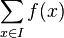$\sum_{x \in I} f(x)$ efficiently for any elementary interval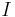$I$, we can compute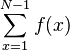$\sum_{x=1}^{N-1} f(x)$ efficiently using the decimal range decomposition. If we are given an arbitrary interval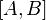$[A, B]$ instead for$x$ to range over, then we just use the fact that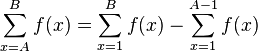$\sum_{x=A}^B f(x) = \sum_{x=1}^{B} f(x) - \sum_{x=1}^{A-1} f(x)$

and use decimal range decomposition on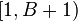$[1, B+1)$ and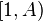$[1, A)$ separately.